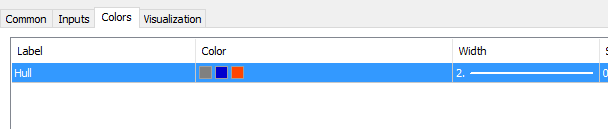# wo to separate Hull MA MT5 buffers

could someone assis to separate the colour buffer here such that  this hull will show 2  buffers  like mt4```//------------------------------------------------------------------
//------------------------------------------------------------------
#property indicator_chart_window
#property indicator_buffers 2
#property indicator_plots   1
#property indicator_label1  "Hull"
#property indicator_type1   DRAW_COLOR_LINE
#property indicator_color1  clrGray,clrMediumBlue,clrOrangeRed
#property indicator_width1  2

//
//
//
//
//

input int                inpPeriod  = 100;          // Period
input double             inpDivisor = 2.0;         // Divisor ("speed")
input ENUM_APPLIED_PRICE inpPrice   = PRICE_CLOSE; // Price

double val[],valc[];

//------------------------------------------------------------------
//
//------------------------------------------------------------------
//
//
//

int OnInit()
{
SetIndexBuffer(0,val,INDICATOR_DATA);
SetIndexBuffer(1,valc,INDICATOR_COLOR_INDEX);
iHull.init(inpPeriod,inpDivisor);
IndicatorSetString(INDICATOR_SHORTNAME,"Hull ("+(string)inpPeriod+")");
return (INIT_SUCCEEDED);
}
void OnDeinit(const int reason)
{
}

//------------------------------------------------------------------
//
//------------------------------------------------------------------
//
//
//
//
//

int OnCalculate(const int rates_total,const int prev_calculated,const datetime &time[],
const double &open[],
const double &high[],
const double &low[],
const double &close[],
const long &tick_volume[],
const long &volume[],
{
int i= prev_calculated-1; if (i<0) i=0; for (; i<rates_total && !_StopFlag; i++)
{
val[i]  = iHull.calculate(getPrice(inpPrice,open,high,low,close,i),i,rates_total);
valc[i] = (i>0) ? (val[i]>val[i-1]) ? 1 : (val[i]<val[i-1]) ? 2 : valc[i-1] : 0;
}
return(i);
}

//------------------------------------------------------------------
// Custom function(s)
//------------------------------------------------------------------
//
//---
//

class CHull
{
private :
int    m_fullPeriod;
int    m_halfPeriod;
int    m_sqrtPeriod;
int    m_arraySize;
double m_weight1;
double m_weight2;
double m_weight3;
struct sHullArrayStruct
{
double value;
double value3;
double wsum1;
double wsum2;
double wsum3;
double lsum1;
double lsum2;
double lsum3;
};
sHullArrayStruct m_array[];

public :
CHull() : m_fullPeriod(1), m_halfPeriod(1), m_sqrtPeriod(1), m_arraySize(-1) {                     }
~CHull()                                                                      { ArrayFree(m_array); }

///
///
///

bool init(int period, double divisor)
{
m_fullPeriod = (int)(period>1 ? period : 1);
m_halfPeriod = (int)(m_fullPeriod>1 ? m_fullPeriod/(divisor>1 ? divisor : 1) : 1);
m_sqrtPeriod = (int) MathSqrt(m_fullPeriod);
m_arraySize  = -1; m_weight1 = m_weight2 = m_weight3 = 1;
return(true);
}

//
//
//

double calculate( double value, int i, int bars)
{
if (m_arraySize<bars) { m_arraySize = ArrayResize(m_array,bars+500); if (m_arraySize<bars) return(0); }

//
//
//

m_array[i].value=value;
if (i>m_fullPeriod)
{
m_array[i].wsum1 = m_array[i-1].wsum1+value*m_halfPeriod-m_array[i-1].lsum1;
m_array[i].lsum1 = m_array[i-1].lsum1+value-m_array[i-m_halfPeriod].value;
m_array[i].wsum2 = m_array[i-1].wsum2+value*m_fullPeriod-m_array[i-1].lsum2;
m_array[i].lsum2 = m_array[i-1].lsum2+value-m_array[i-m_fullPeriod].value;
}
else
{
m_array[i].wsum1 = m_array[i].wsum2 =
m_array[i].lsum1 = m_array[i].lsum2 = m_weight1 = m_weight2 = 0;
for(int k=0, w1=m_halfPeriod, w2=m_fullPeriod; w2>0 && i>=k; k++, w1--, w2--)
{
if (w1>0)
{
m_array[i].wsum1 += m_array[i-k].value*w1;
m_array[i].lsum1 += m_array[i-k].value;
m_weight1        += w1;
}
m_array[i].wsum2 += m_array[i-k].value*w2;
m_array[i].lsum2 += m_array[i-k].value;
m_weight2        += w2;
}
}
m_array[i].value3=2.0*m_array[i].wsum1/m_weight1-m_array[i].wsum2/m_weight2;

//
//---
//

if (i>m_sqrtPeriod)
{
m_array[i].wsum3 = m_array[i-1].wsum3+m_array[i].value3*m_sqrtPeriod-m_array[i-1].lsum3;
m_array[i].lsum3 = m_array[i-1].lsum3+m_array[i].value3-m_array[i-m_sqrtPeriod].value3;
}
else
{
m_array[i].wsum3 =
m_array[i].lsum3 = m_weight3 = 0;
for(int k=0, w3=m_sqrtPeriod; w3>0 && i>=k; k++, w3--)
{
m_array[i].wsum3 += m_array[i-k].value3*w3;
m_array[i].lsum3 += m_array[i-k].value3;
m_weight3        += w3;
}
}
return(m_array[i].wsum3/m_weight3);
}
};
CHull iHull;

//
//---
//

template <typename T>
double getPrice(ENUM_APPLIED_PRICE tprice, T& open[], T& high[], T& low[], T& close[], int i)
{
switch(tprice)
{
case PRICE_CLOSE:     return(close[i]);
case PRICE_OPEN:      return(open[i]);
case PRICE_HIGH:      return(high[i]);
case PRICE_LOW:       return(low[i]);
case PRICE_MEDIAN:    return((high[i]+low[i])/2.0);
case PRICE_TYPICAL:   return((high[i]+low[i]+close[i])/3.0);
case PRICE_WEIGHTED:  return((high[i]+low[i]+close[i]+close[i])/4.0);
}
return(0);
}
//------------------------------------------------------------------
```
Reason: